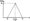# Information Theory and Coding July 2011

UNIT-I

1.(a) Define Entropy. Show that the entropy is maximum when all the messages are equiprobable. Assume M = 3.

(b) A Random Variable has a density function as shown below. Find the corresponding entropy.OR

2. (a) In a message conveyed through a long sequence of dots and dashes, the probability of occurrence of a dash is one third of that of a dot. the duration of a dash is three times that of a dot. If the dot lasts for 10 m sec. and the same time is allowed between symbols, determine the following :

(i)  The information in dot and dash

(ii)  Average information in the dot-dash code

(iii) Average information rate.

(b) Give differences between Discrete and Continuous communication channel.

UNIT II

3. (a) State Shannon Hartley Theorem. Give its implications.

(b) Define Transformation. Prove that the transinformation of a continuous system is non-negative.

OR

4. (a) Prove that the channel capacity of a white-bandlimited Gaussian channel is C = w log f 1 it i s,

N. where w = Channel Bandwidth S/N = Signal to Noise Ratio.

(b) A Gaussian channel has 1 MHz bandwidth. Calculate the channel capacity if its signal power to (two sided) noise spectral density ratio is 5 x 104 Hz. Also find the maximum information rate.

UNIT III

5  (a) State Kraft’s inequality.

(b) Define the following terms :

(i) Source coding

(ii) Channel coding

(iv) Entropy coding.

(c)  Explain Error Control coding with the help of a suitable diagram.

6 (a) Explain the following with suitable example :

(1) Variable Length Code

(ii) Prefix Free Code

(iii) Uniquely Decodable Code

(v)  Instantaneous Code.

(b) Explain ARQ and FEC methods of Error Control Coding. List their advantages and disadvantages.

UNIT IV

7  (a) Given a (7,4) code with g(x) = x3 + x2 + l, construct the decoding table for this single error correcting code.

(b) Determine the data vector transmitted for the received vector, r = 1101101

OR

8. Consider the following (k+1, k) systematic LBC, with the parity check digit, C^+^ given by C, = d. © d~ ©© d,. A’+l I 2   k

(a)  Construct appropriate generator matrix for this code.

(b)  Construct the code generated by this matrix for k = 3.

(c) Determine the error correcting and error detecting capability of this code.

(d) Show that CHt = 0 and rH = 0 if no error occurs 1 if error occurs

UNIT – V

9 (a) Compare coded and uncoded systems in terms of Probability of Error

(b) Write short note on :

(i) Interlaced Code

(ii) Sequential Coding.

OR

10 (a) For the convolutional Encoder shown below

(i)  Draw the state diagram

(ii) Draw the trellis diagram

(iii) Determine the o/p sequence for the input data, d = 11010100.